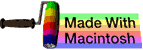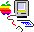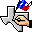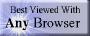music# order of precedence

## summary

This subchapter looks at order of precedence.

## free computer programming text book project

If you like the idea of this project,# order of precedence

This subchapter looks at order of precedence.

Every programming language needs to have some system for determining the order of evaluation of expressions.

x := 5 + 6 * 7;
or
x = 5 + 6 * 7

Is x 47 or 77?

(5 + 6) = 11; followed by 11 * 7 = 77

(6 * 7) = 42; 5 + 42 = 47

The normal rules of elementary algebra call for multiplication (and division) to have a higher priority than addition (or subtraction), which would make x = 42.

elementary algebra
parenthesis
exponentiation
multiplication
division
subtraction

A strict left to right evaluation would make the answer x = 77.

A strict right to left evaluation would make the answer x = 42.

The use of a reverse Polish notation (RPN, such as is used in Forth) avoids the question.

The two most common approaches used in computer programming languages are either (1) some order of precendence) or (2) directional evaluation (left-to-right or right-to-left).

The use of parenthesis can be used to change the normal order of evaluation.

Parenthesis can also be used to make complicated expressions more clear to a human reader. This makes the program easier to udnerstand and easier to maintain over years or decades of use.

The order of precedence in the following charts are from highest to lowest (top to bottom). Items on the same level are of equal order of precendence.

## C

Primary-expression operators
15( )    [ ]    .    ->
Unary operators
14*    &    -    !    ~    ++    --    sizeof (type)
13*    /    %
12+    -
11>>    <<
10<    >    <=    >=
9==    !=
8&
7^
6|
5&&
4||
3?:
Assignment operators
2=    +=     -=    *=    /=    %=
>>    =<    <=    &=    ^=    |=
Sequence operator
1, (comma)

## Pascal

 unary +    unary -    not *    /    div    mod    and +    -    or =    <>    <    <=    >    >=    in

## PL/I

 1 all subexpressions in parenthesisfrom innermost to outermost 2 all exponentiationsfrom right to left 3 all multiplications and divisionsfrom left to right 4 all additions and subtractionsfrom left to right

## ALGOL

 1 parenthesis 2 ↑ (exponentiation) 3 ×    /    ÷(multiplication and division) 4 +    -(addition and subtraction) 5 <    ≤    =    ≠    ≥    >(relational operators) 6 ¬ (not) 7 ∧ (and) 8 ∨ (or) 9 ⊃ (implies) 10 ≡ (is equivalent to)

Note that the actual ALGOL symbol for is not greater than has the less than symbol over the equals symbol rather than ≤ and the ALGOL symbol for not less than has the greater than symbol over the equals symbol rather than ≥.

## JOVIAL

The following material is from the unclassified Computer Programming Manual for the JOVIAL (J73) Language, RADC-TR-81-143, Final Technical Report of June 1981.

1.1.3 Calculations

In the simplest case, calculations is performed by an assignment
statement.  An example is:

AVERAGE = (X1 + X2)/2;

The right-hand-side of this assigment is a formula; it forms the
sum of X1 and X2 and divides it by 2.  The details of the
operation depend on how X1 and X2 are declared.  If X1 and X2 are
declared float, the calculation is very likely to produce the
expected result.  In contrast, if the X1 and X2 are declared
fixed, the scaling must be worked out by the programmer to make
sure the calculation will succeed.  And if X1 and X2 are declared
character-string, the compiler will reject it because JOVIAL does
not automatically convert values into the types required by
operators.

In the example just given, the parenthesis show that the addition
is performed before the division.  When parenthesis are not
given, JOVIAL recognizes the usual order of evaluation.  Here is
an example:

POLY = BETA*X1**2 - GAMMA*X2 + DELTA;

JOVIAL applies its "rules of precedence" to the formula in this
assignment and thus interprets it as:

POLY = (((BETA*(X1**2)) - (GAMMA*X2)) + DELTA);

The complete precedence rules are given in Chapter 11.

Chapter 1 Introduction, page 6

# free music player coding example

Coding example: I am making heavily documented and explained open source code for a method to play music for free — almost any song, no subscription fees, no download costs, no advertisements, all completely legal. This is done by building a front-end to YouTube (which checks the copyright permissions for you).

View music player in action: www.musicinpublic.com/.

Create your own copy from the original source code/ (presented for learning programming).

## view text bookHTML file

Because I no longer have the computer and software to make PDFs, the book is available as an HTML file, which you can convert into a PDF.

 Tweets by @osdata

## free computer programming text book project

Building a free downloadable text book on computer programming for university, college, community college, and high school classes in computer programming.

If you like the idea of this project,

send donations to:
Milo
PO Box 1361
Tustin, California 92781

Supporting the entire project:

If you have a business or organization that can support the entire cost of this project, please contact Pr Ntr Kmt (my church)

Some or all of the material on this web page appears in theThis web site handcrafted on Macintoshcomputers using Tom Bender’s Tex-Edit Plusand served using FreeBSD.†UNIX used as a generic term unless specifically used as a trademark (such as in the phrase “UNIX certified”). UNIX is a registered trademark in the United States and other countries, licensed exclusively through X/Open Company Ltd.

Names and logos of various OSs are trademarks of their respective owners.